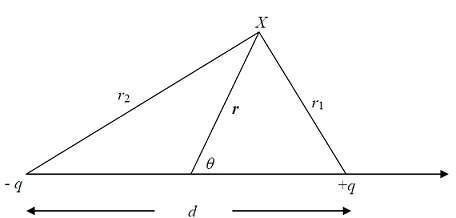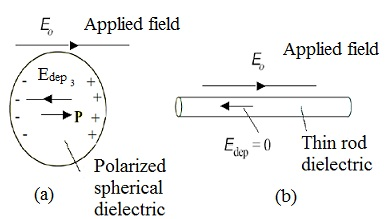#### Dielectric Properties of Solids, Physics tutorial

Introduction:

Solid state physics is a study of crystalline solids, through methods like quantum mechanics, electromagnetism, crystallography, and metallurgy. It is largest branch of condensed matter physics. Solid state physics studies how large-scale properties of solid materials result from atomic-scale properties. Therefore, solid state physics forms theoretical basis of material science. It has direct applications, for instance in the technology of transistors and semiconductors. Solid materials can be separated into two distinct groups on the basis of their behaviour under influence of the external electric field.

Conductors - those in which there are electrons that are free to move in presence of field. Examples of these are metals, carbon, etc.

ii. Insulators or dielectrics - those in which electrons are strongly bound to atoms or molecules composing material and can't be detached by application of the electric field to the materials. Examples are sulphur, porcelain, mica, etc.

Dielectric Properties:

The dielectric is a non-conducting material, like glass, rubber, or waxed paper. The following are some of the properties of dielectric:

i) When the dielectric material is inserted between plates of capacitor, the capacitance increases by factor K, known as dielectric constant of dielectric

C = KC0

Where C0 is capacitance in absence of dielectric

ii) the dielectric constant K has no unit and it is characteristic of given material

iii) If εo is permittivity of free space and ε is permittivity of dielectric material, therefore

K = ε/ε0

iv) With introduction of dielectric materials, energy density becomes

u = 1/2εE2

v) Potential difference V between plates when the dielectric material is inserted, given that charge remains unchanged, is provided as:

V = V0/K

Where Vo is potential difference when dielectric is not inserted. Equation defines that potential difference V between plates decreases by the factor of K.

vi) If potential difference is kept unchanged when dielectric material is inserted, then we have,

Q = KQ0

Therefore, charge on the capacitor with fixed potential difference between its plates is increased by factor K

vii) If electric field between parallel plate capacitor is Eo and it is E after material with dielectric constant K is inserted into space between plates, then

E = E0/K

The electric field within dielectric is reduced by factor equal to dielectric constant

viii) Net field E within dielectric is provided as

E = E0 - Eind

Eind = E0(1 - 1/K)

ix) The induced electric field in dielectric is related to induced charge density σind through relationship Eind = σind0

x) σind = σ(1 - 1/K) and Qind = Q(1 - 1/K)

As K is always greater than 1, these expressions show that Eind > Eo, σind > σ and Qind > Q.

Local Electric Field:

It must be noted that:

• every material is composed of very large number of atoms/molecules
• Atom comprises of positively charged nucleus and negatively charged electrons
• System comprising of two equal and opposite charges q, separated by the certain distance d, is electric dipole
• When the atom or molecule of dielectric is placed in electric field, positive and negative charges feel opposite forces and are separated forming a dipole.

Assume the charges -q and +q are placed, respectively, at d/2 and -d/2 from origin, as shown below. Magnitude of potential because of this system at a point X is provided by

Φ(r) = q/4πε0(1/r1 - 1/r2)

Where r1, r2 are distances of X from + q and - q respectively.If θ is angle between axis of charges and position vector of X, we can write

1/r1 ≈ 1/r + dcosθ/2r2 and 1/r2 ≈ 1/r - dcosθ/2r2

After solving:

Φ(r)  = (q/4πε0)(dcosθ/r2)

Dipole moment, p, is vector along axis of dipole pointing in direction - q to + q and having magnitude qd. In terms of dipole moment, Equation becomes:

Φ(r) = pcosθ/4πε0r2 = p‾.r‾/4πε0|r|3

Φ(r) = -P‾/4πε0r2 . ∇(1/r) = -p‾.∇Φ0

Φ0 is potential of unit charge.

Field of electric dipole may also be stated in following way,

E‾ = 1/4πε0[(3(p‾.r‾)r‾ - r2p‾)/r5]

Considering cubic crystal dielectric and suppose electric field of magnitude Eo is applied parallel to one of axes of crystal. Field will push positively charged nucleus of atoms of dielectric slightly in direction of field and negatively charged electrons in opposite direction. We say that atom is polarized under influence of applied external field and charges are called polarization charges. The polarization P is stated as dipole moment p per unit volume, averaged over volume of the cell. Total dipole moment is stated as

P‾ = Σqnrn

Let us imagine that small sphere is cut out of specimen around reference point; then E1 is field of polarization charges on inside of cavity left by sphere, and E2 is field of atoms within cavity. Polarization charges on outer surface of specimen produce depolarization field, E3. Depolarization field is opposite to P, that is, field E3 is known as depolarization field, as within body it tends to oppose applied field Eo. The dielectric obtains polarization because of applied electric field Eo. This polarization is result of redistribution of charges inside dielectric.

The local electric field Eloc at any atom of dielectric may be expressed as

Eloc = E0 + E1 + E2 + E3

Contribution E1 + E2 + E3 is total effect at one atom of dipole moments of all the other atoms in system (in CGS units):

E1 + E2 + E3 = (3(p‾.r‾)r‾ - r2p)/r5 and in SI unit E1 + E2 + E3 = 1/4πε0[(3(p‾.r‾)r‾ - r2p)/r5]If Px, Py, Pz are the components of the polarisation P referred to the principal axes of an ellipsoid, then the components of the depolarization field are written

E3x = -NxPx0; E3y = -NyPy0; E3Z = -NzPz0

Dielectric Constant and Polarizability:

Consider dielectric behaviour of molecules that have permanent dipole moment. Dielectric constant ε has been expressed for isotropic or cubic medium relative to vacuum as:

ε = D/E = 1 + P/ε0E = 1+χ

Where χ is electric susceptibility.

Susceptibility (in SI unit) is related to dielectric constant by

χ = P/ε0E = ε - 1

If field is not too large, strength of induced dipole moment in the atom i is proportional to local electric field acting on dielectric. That is

Pi ∝ Eloc = Pi = αiEiloc

Where αi is Polarizability and pi is dipole moment of atom i.

Electric susceptibility can now be expressed as:

χ = P/E = ΣiEilocNiαi/Eloc - (4π/3)P

Using Lorentz relation,

P/E = ε - 1/4π

Therefore (in CGS) ΣNiαi = 3/4π((ε - 1)/(ε + 2))

This equation is called as Clausius-Mossotti equation, defines relation between dielectric constant and atomic polarizabilities

Dipole Relaxation and Dielectric Losses:

Dipole relaxation time is time interval characterizing restoration of disturbed system to equilibrium configuration; the relaxation frequency is stated as reciprocal of relaxation time. Orientational contribution to dielectric constant is the major cause to difference between low frequency dielectric constant and high frequency dielectric constant. Orientational relaxation frequencies are strongly dependent on temperature and frequency as:

α = α0/(1 + iωτ)

Where τ is called as Debye relaxation time, α0 is static orientational Polarizability and ω is angular frequency.

Tutorsglobe: A way to secure high grade in your curriculum (Online Tutoring)